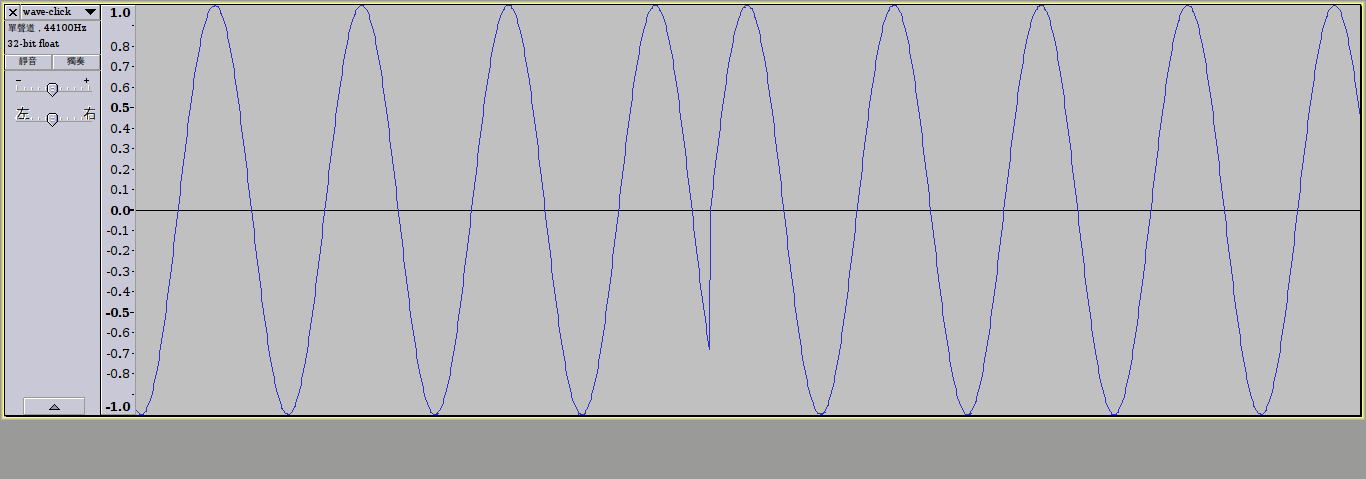```<CsoundSynthesizer>
<CsOptions>
</CsOptions>

<CsInstruments>
sr = 44100   ;sample rate
ksmps = 1    ;samples in a control period
nchnls = 1   ;number of channels
0dbfs = 100  ;value of 0db

instr 1
;p4=freq
;p3=duration
iamp = ampdb(p4)        ;convert decibels to linear amp
ifreq = cpspch(p4)      ;convert pitch to frequent

a1 oscil 100, ifreq, 1  ;oscillate f1
out a1                  ;output
endin
</CsInstruments>
<CsScore>
f1 0 16384 10 1
t 0 60

;ins	strt	dur	pitch
i1	0	1	8.00
i1	+	1	8.00
i1	+	1	8.00
i1	+	1	8.00

e
</CsScore>
</CsoundSynthesizer>``````<CsoundSynthesizer>
<CsOptions>
</CsOptions>

<CsInstruments>
sr = 44100   ;sample rate
ksmps = 1    ;samples in a control period
nchnls = 1   ;number of channels
0dbfs = 100  ;value of 0db

instr 1
;p4=freq
;p3=duration
iamp = ampdb(p4)        ;convert decibels to linear amp
ifreq = cpspch(p4)      ;convert pitch to frequent

;Modify freq to avoid click

a1 oscil 100, ifreq, 1  ;oscillate f1
out a1                  ;output
endin

</CsInstruments>
<CsScore>
f1 0 16384 10 1
t 0 60

;ins	strt	dur	pitch
i1	0	1	8.00
i1	+	1	8.00
i1	+	1	8.00
i1	+	1	8.00

e
</CsScore>
</CsoundSynthesizer>```